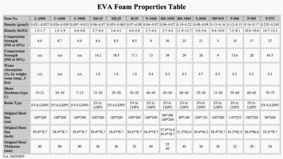### DENSITY

Density, is the mass (weight) of a substance per unit volume. The commonly used units are grams per cubic centimeter (g/cm^3), kilograms per cubic meter (kg/m^3) or pounds per cubic feet (lb/ft^3).
Calculation:

D = m / v

where:
D = density, kg/m^3 (g/cm^3, or lb/ft^3).
m = mass, kg (g, or lb).
v = volume, m^3 (cm^3, or ft^3).More technical data of EVA foam material